Table of ContentOpen Access

ARTICLE

Qiang Li1,2,*, Pan Chen1, Lihong Gao1, Dan Meng1, Jinjie Zou1
1 Huadong Engineering (Shenzhen) Corporation Limited, Shenzhen, 518100, China
2 Faculty of Civil Engineering and Geosciences, Delft University of Technology, Delft, 2628 CN, The Netherlands
* Corresponding Author: Qiang Li. Email:(This article belongs to this Special Issue: Computer Modeling in Ocean Engineering Structure and Mechanical Equipment)

Computer Modeling in Engineering & Sciences 2023, 135(1), 465-481. https://doi.org/10.32604/cmes.2022.020300

Received 16 November 2021; Accepted 13 May 2022; Issue published 29 September 2022

### Keywords

List of Notation

1  Introduction

As a result of low-carbon green growth policies, many countries have mandated an increase in renewable energy production. The international market for renewable energy is therefore growing steadily each year. Among various clean and renewable energy sources, wind energy has proved particularly attractive , equivalent to approximately 199 GW. The market is rapidly expanding in many countries, including the United States, Northern Europe, Japan, China and Korea . Based on the historical data from 1980 to 2010 and combined with data forecast for the period of 2010 to 2050, wind energy is estimated to meet more than 20% of the world's electricity demand by mid-century .

Of all the foundations, monopiles are the most preferred [4,5], accounting for more than 87% of the total installations by 2018 . As a supporting substructure of offshore wind turbines, monopiles are required to resist both the vertical load from self-weight and the lateral loads from wind, wave and currents. To date, in view of relatively small vertical load, monopile design is dominated by lateral loads. However, due to increased turbine size (larger vertical load from self-weight) and hub heights (higher lateral loads from wind) for larger electric capacity, monopile response under combined load has attracted industrial interest.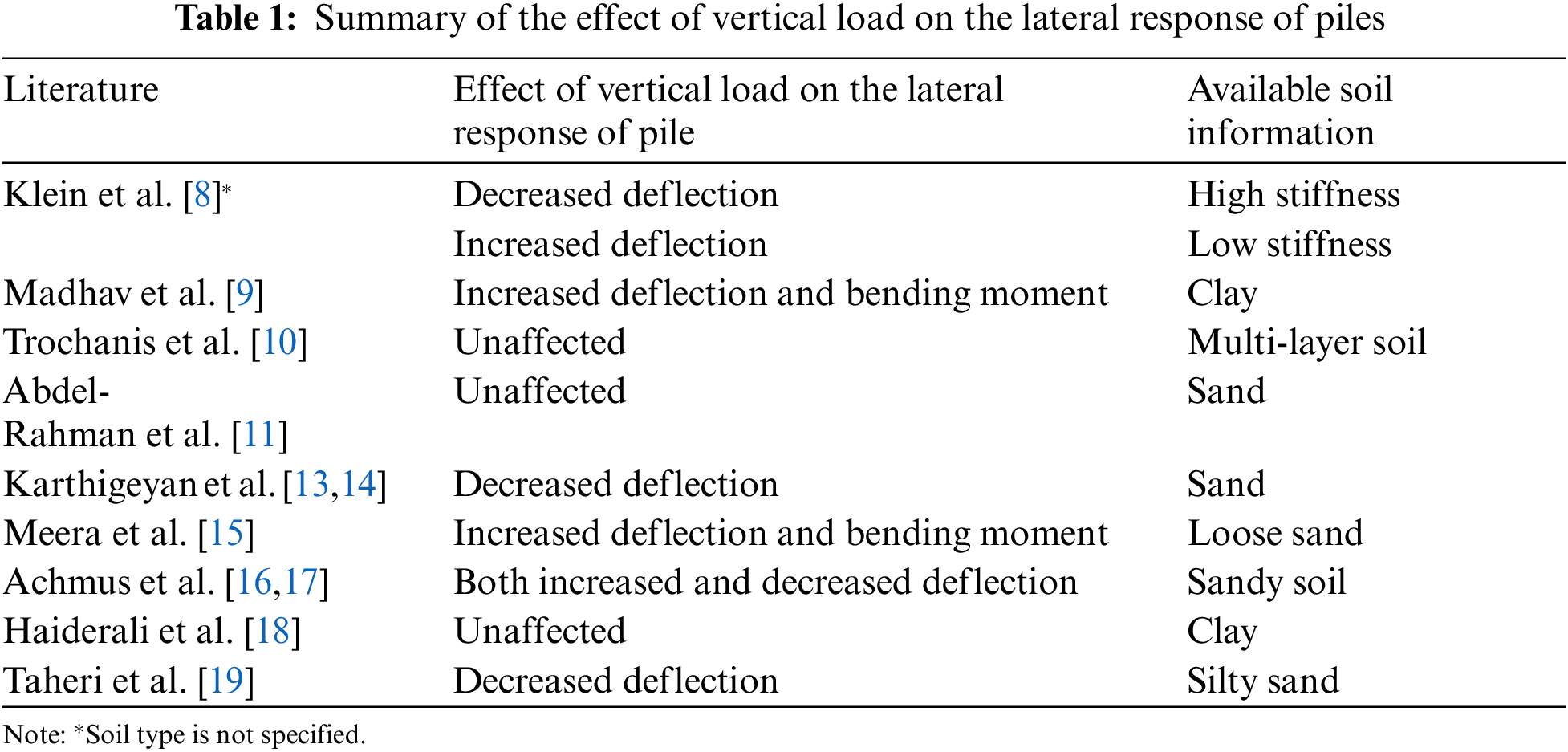This paper numerically studied the effect of vertical load on the lateral response of open-ended monopiles in the sand. The influence of load application sequence, load magnitude and pile slenderness ratio (L/D; L, pile length, and D, pile diameter) was investigated. PLAXIS 3D  was used for the FEA. In all cases considered, the pile was embedded in homogeneous dry sand and was assumed to be fully drained . More details about the simulations can be found in Section 2.

2  Finite-Element Modelling

A typical three-dimensional finite element mesh of pile-soil system used in this study is shown in Fig. 1. Considering the symmetry of the problem, only half of the pile-soil system was modelled to improve computation efficiency. The model mesh was 40D long and 20D wide. The model was 20D beneath the pile tip. As can be seen from Fig. 1, the mesh around the pile was highly refined. All the nodes on the lateral boundaries were restrained in the normal direction, while the bottom surface was completely fixed in all three directions. Additional simulation using a model of double size and mesh density exhibited little change in the lateral load-deflection response of pile (less than 2%), which justified the accuracy of the model adopted in Fig. 1.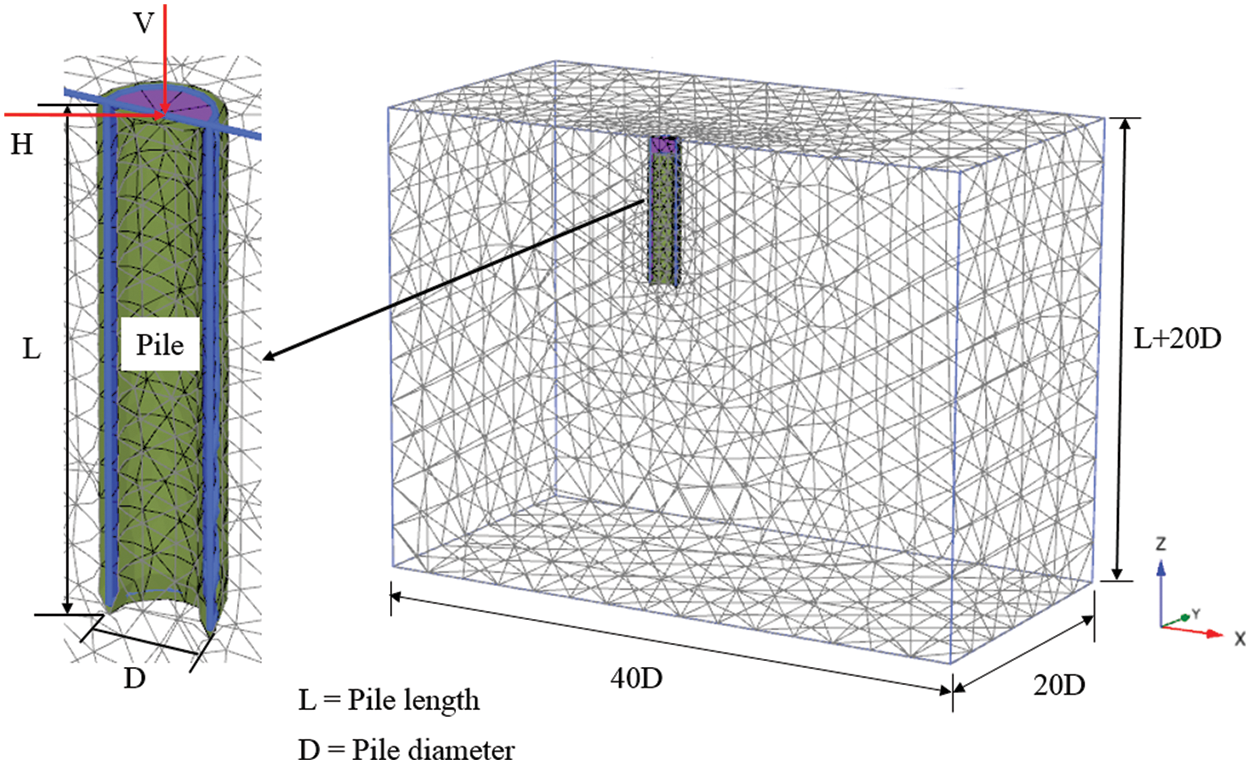Figure 1: Typical mesh adopted in three-dimensional finite element analyses

A series of analyses were performed on a single free-headed pile in sand. The material behaviour of the piles was assumed to be linearly elastic with the parameters E = 210 GPa (Young’s modulus) and ν = 0.2 (Poisson’s ratio) for steel. The pile geometries considered are summarised in Table 2. The Hardening-Soil (HS) model was employed to describe the nonlinear behaviour of dense sand.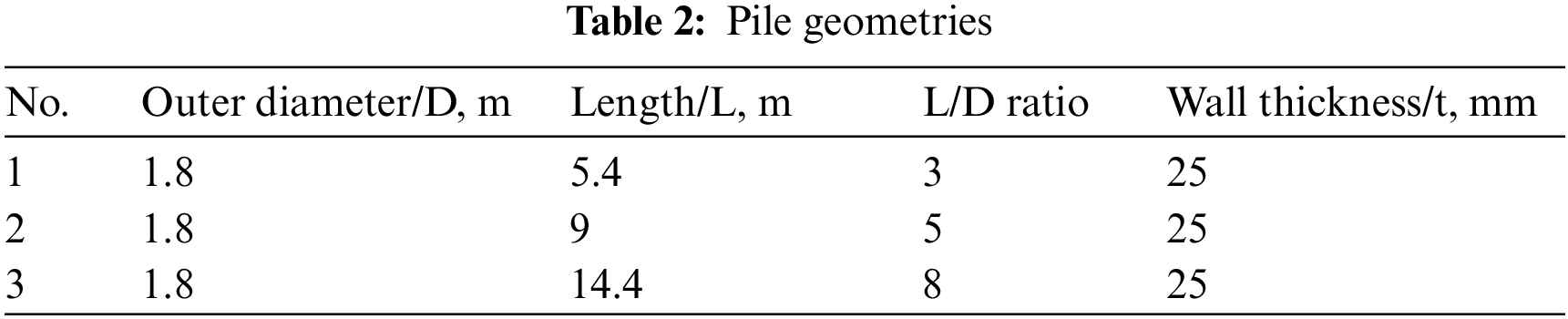The Hardening-Soil model contained eight parameters, including three basic parameters for soil stiffness [20,21]:

m, power for stress level dependency of stiffness,

pref, reference stress for stiffness,

K0NC, K0 value for normal consolidation and

Rf, failure ratio qf/qa.

The Harding-Soil model assumed a hyperbolic relationship between vertical strain ϵ1 and deviatoric stress q in primary triaxial loading, compared with the bi-linear curve in Mohr-Coulomb model. In addition, the model also captured the stress level dependency behaviour of sand. In this regard, the secant stiffness modulus at half of shear strength (E50), the elastic unloading modulus (Eur) and the oedometer modulus (Eoed) in Hardening-Soil model were independently defined by a reference minor principal stress of σ3=σref for Eoed, as shown in Eq. (1). The E50ref, Eurref and Eoedref needed to be defined. According to the Hook’s theory, shear modulus (Gur) was calculated by Eur=2(1+ν)Gur.

Eoed=Eoedref(σ1+ccotφpσref+ccotφp)m(1)

More details about the HS model and the calibration of model parameters can be found in Schanz et al.  and Brinkgreve et al. . The parameters employed in this paper were derived on the basis of an assumed relative density of sand (80%) using the empirical formulas recommended by Brinkgreve et al. .

In order to apply load at the central point of the pile cross-section, the pile top was closed by a steel plate with a thickness of 50 cm, making it sufficiently rigid and preventing obvious deformation during loading. The sand inside the pile was removed to 1 m below the original mudline to eliminate the possibility of extra pile resistance.

The finite element calculations were executed in several phases. Firstly, initial stress state in the system caused by the self-weight of the soil was generated using soil elements only. Subsequently, pile elements were generated by assuming a ‘wished in place’ state, i.e., the installation process of the pile was not modelled. The soil elements within 1 m from the original mudline inside the pile were removed. The various loading stages were specified in PLAXIS, more details of which are provided in the subsequent sections.

3  Analysis and Results

The analysis results of piles under combined load are given in this section. Section 3.1 investigates how the lateral response characteristics of pile vary with load application sequence, while Section 3.2 quantifies the influence of pile slenderness on pile response. Section 3.3 further reveals the underlying mechanism that governs the combined loading response of monopiles by investigating soil stress state around the pile.

3.1 Influence of Load Application Sequence on Pile Response

Influence of vertical load on pile lateral response was investigated considering different load application sequences and varying load magnitudes. Monopiles with a diameter of 1.8 m and lengths of 5.4, 9 and 14.4 m, were respectively studied, as presented in Tables 2 and 3 with full modelling properties employed. The vertical load was applied to a pile in three different sequences (see Fig. 2), (i) prior to lateral load (VPL, Fig. 2a), (ii) simultaneously with lateral load (SAVL, Fig. 2b), and (iii) after lateral load (LPV, Fig. 2c). The deflection of pile top was extracted from the central node on the head plate of the pile model.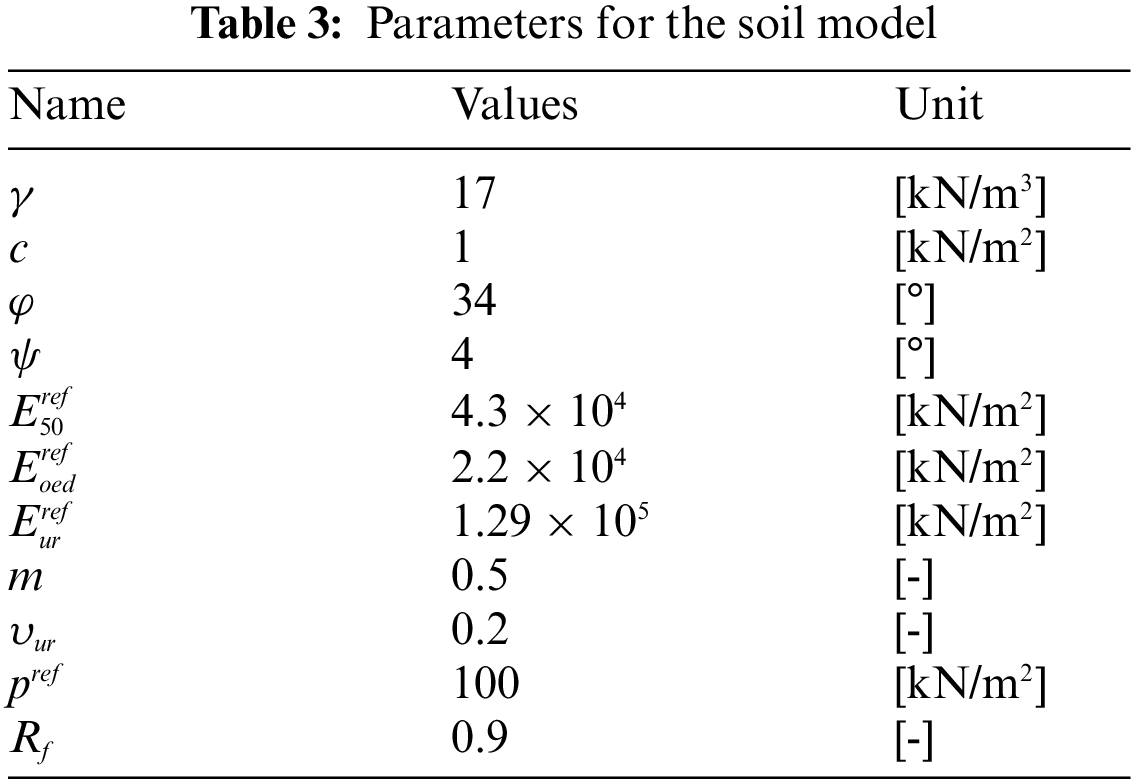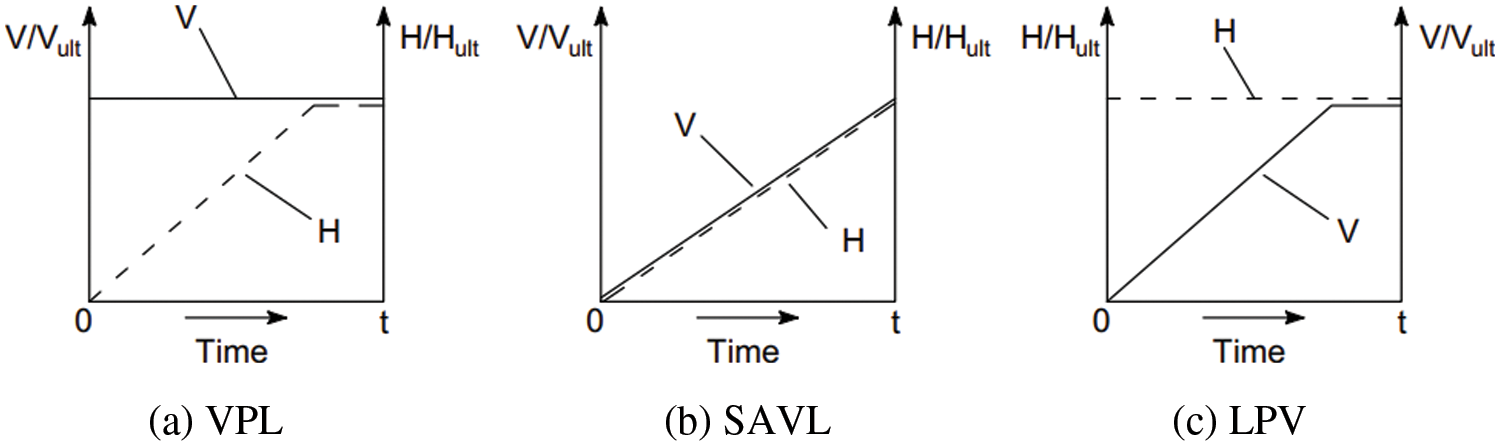Figure 2: Schematic illustration of load sequence (a) VPL (b) SAVL (c) LPV

Firstly, the vertical load capacity (Vult) and lateral load capacity (Hult) of a single pile were separately evaluated in numerical analyses. The loads were applied incrementally in two directions to the pile up to a displacement of 0.1D (0.18 m). As shown in Fig. 3a, Vult for piles with L/D ratios of 3, 5 and 8 were calculated as 2800, 4750 and 8100 kN, respectively. Meanwhile, as shown in Fig. 3b, Hult for piles with L/D ratios of 3, 5 and 8 were calculated to be 680, 1450 and 2650 kN, respectively.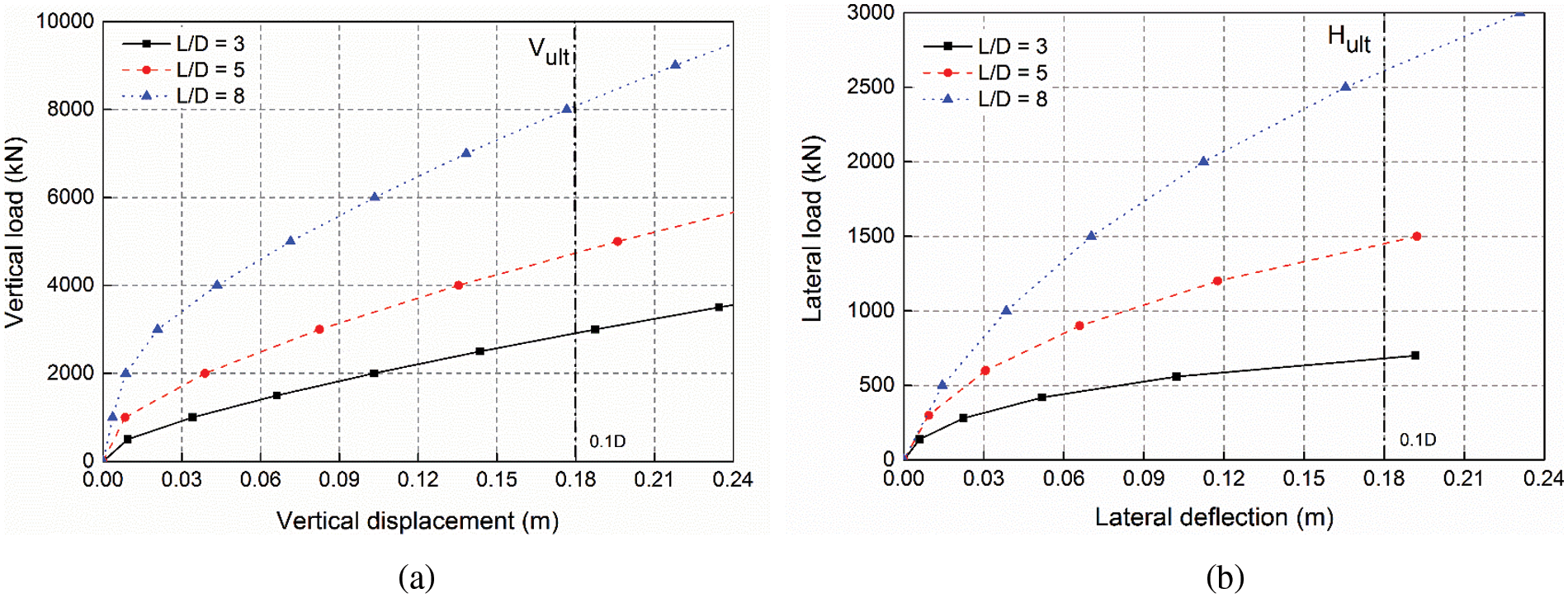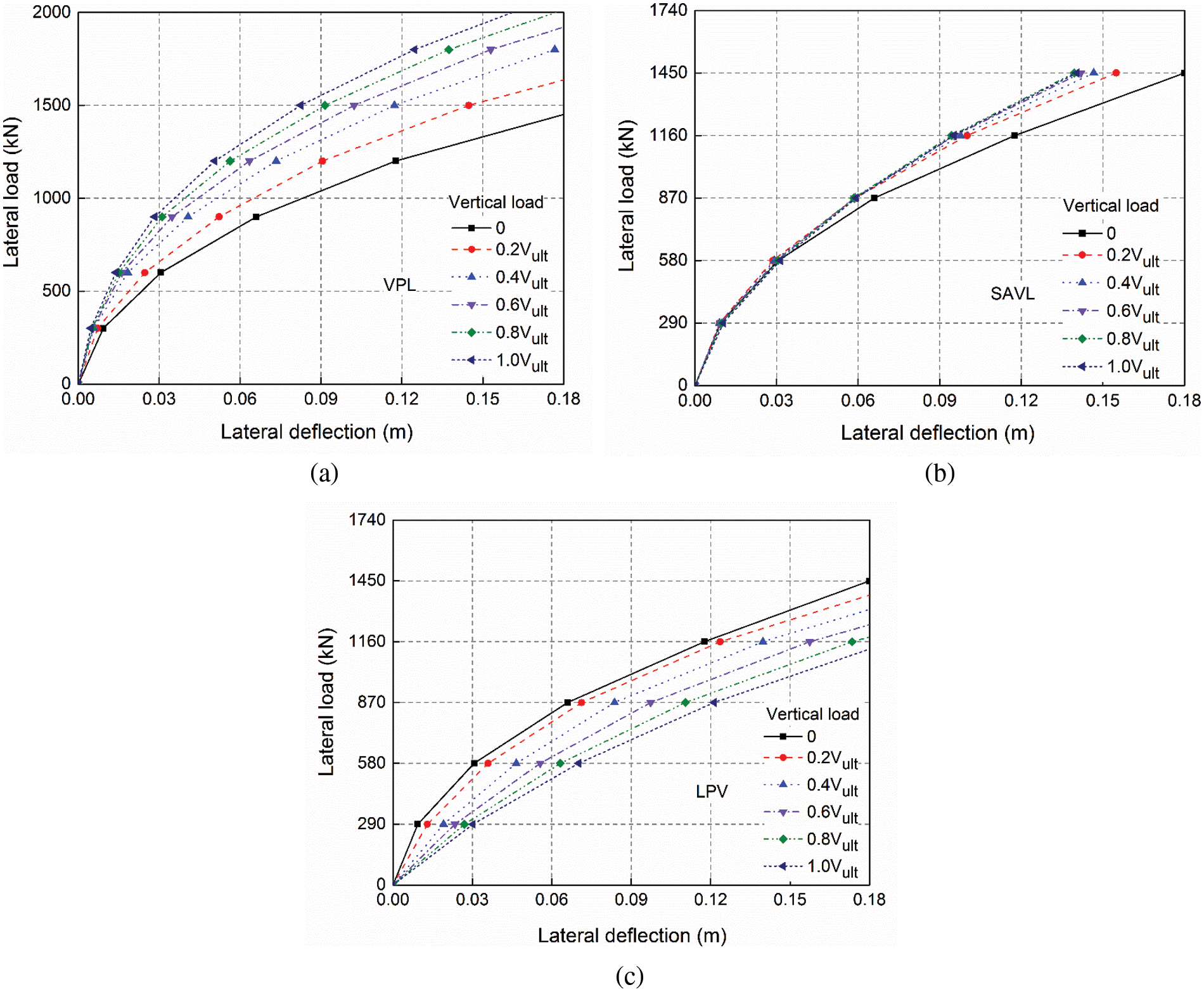Figure 4: Lateral load-deflection behaviour for cases (L/D = 5): (a) VPL, (b) SAVL, and (c) LPVFigure 5: (a) Comparison of lateral load-deflection behaviour, (b) Change of pile lateral capacity by vertical loading

Fig. 5a compares the lateral load-deflection behaviour of the pile under a given vertical load of 0.6 Vult in the VPL, SAVL and LPV cases. Under the condition of the same vertical and lateral load, the lateral deflection of the pile was the largest in the LPV case, successively followed by those in the SAVL and VPL cases. Fig. 5b shows the change in the ultimate lateral capacity of the pile with the increase of vertical load in the VPL, SAVL and LPV cases. It can be seen from the figure that with the increase of pile capacity, vertical load demonstrated a favourable effect in the VPL and SAVL cases. For instance, as vertical load increased, lateral capacity considerably increased in the VPL case (in the order of 15% to 42%) and increases marginally in the SAVL case (in the order of 12% to 30%). However, lateral capacity marginally decreased in the LPV case (in the order of −0.4% to −10%).

3.2 Influence of Slenderness Ratio (L/D) on Pile Response

In practical applications, piles used for wind turbine have various embedment depths, depending on the sea water depth, marine sand properties, loading conditions, and surrounding hydrodynamic environments, among other factors . The influence of vertical load on the lateral response of piles considering different pile slenderness (L/D ratio) should be an important factor to consider during design. The numerical results discussed above are based on pile with an L/D ratio of 5. In the study herein, piles with L/D ratios of 3, 5 and 8 were investigated and compared. L/D ratios were evaluated by keeping pile diameter constant while varying pile length.

Fig. 6 presents the simulation results of the influence of load application sequence on the pile lateral deflection in the VPL, SAVL and LPV cases, respectively. As shown in Fig. 6a, the lateral deflection of the pile decreased with the increase of vertical load in the VPL case. Pile with smaller L/D ratio experienced a more significant decline in the lateral deflection under the same proportion of the vertical load. At the ultimate vertical load magnitude (Vult), the pile with an L/D ratio of 3 witnessed a reduction on lateral deflection by around 75% relative to the case where no vertical load was applied. However, with the increase of aspect ratio, the lateral deflection of the pile with aspect ratios of 5 and 8 respectively decreased by 56% and 35%.Figure 6: Influence of L/D ratio on the pile lateral deflection for (a) VPL case, (b) SAVL case and (c) LPV case considering different load application sequences

As can be seen from Fig. 6b, the lateral deflection of the pile decreased with the increase of vertical load in the SAVL case, but less significantly than that in the VPL case. The most significant decrease in the lateral deflection occurred when vertical load increased from 0 to 0.2 Vult., showing that the smaller the L/D ratio, the more significantly the vertical load reduced the lateral displacement of the pile. At the vertical load level of Vult, pile with an L/D ratio of 3 experienced a decline in the lateral deflection by around 50%, compared with the 27% and 7% for the piles with aspect ratios of 5 and 8, respectively.

It can be seen from Fig. 6c that the lateral deflection of the pile increased with vertical load at a constant rate in the LPV case. And it also shows that the smaller the L/D, the greater the increase of the lateral deflection under the the same proportion of vertical load. Piles with L/D ratios of 3 and 5 shared a similar trend in the increase of lateral deflection, slightly higher than that with an L/D ratio of 8. At the vertical load level of Vult, the lateral deflection of the piles with L/D ratios of 3 and 5 increased by approximately 42%, while that of the pile with an L/D ratio of 8 increased by 35%.

From the analysis above, it can be concluded that piles with smaller embedment depth were more sensitive to the change of vertical load amplitude. In the VPL and SAVL cases, the applied vertical load could produce confining effect on the surrounding sand, leading to an increase in sand stiffness while reducing lateral deflection and rotation thereafter. In the LPV case, extra lateral deflection would be induced by the applied vertical load due to the p-Δ effect.

3.3 The Underlying Effect Mechanism of Vertical Load on the Combined Loaded Monopiles in the VPL Case

The above analyses clearly indicate that the sequence of load application governs the lateral response characteristics of pile under combined load. In the offshore wind turbine projects, L/D ratio is generally less than 5, and VPL case is the most commonly seen in practice. The preceding discussion has proved that vertical load has a favorable effect on the lateral response of pile in the VPL case. To reveal its underlying mechanism, the variations of mean effective stresses and lateral effective stresses along the depth of the piles were extracted, as presented in Fig. 7 . The locations of selected stress points are illustrated in Fig. 8. Stress points were located in front of the pile (at a distance of 0.01 m), relative to the loading direction, as shown in the figure.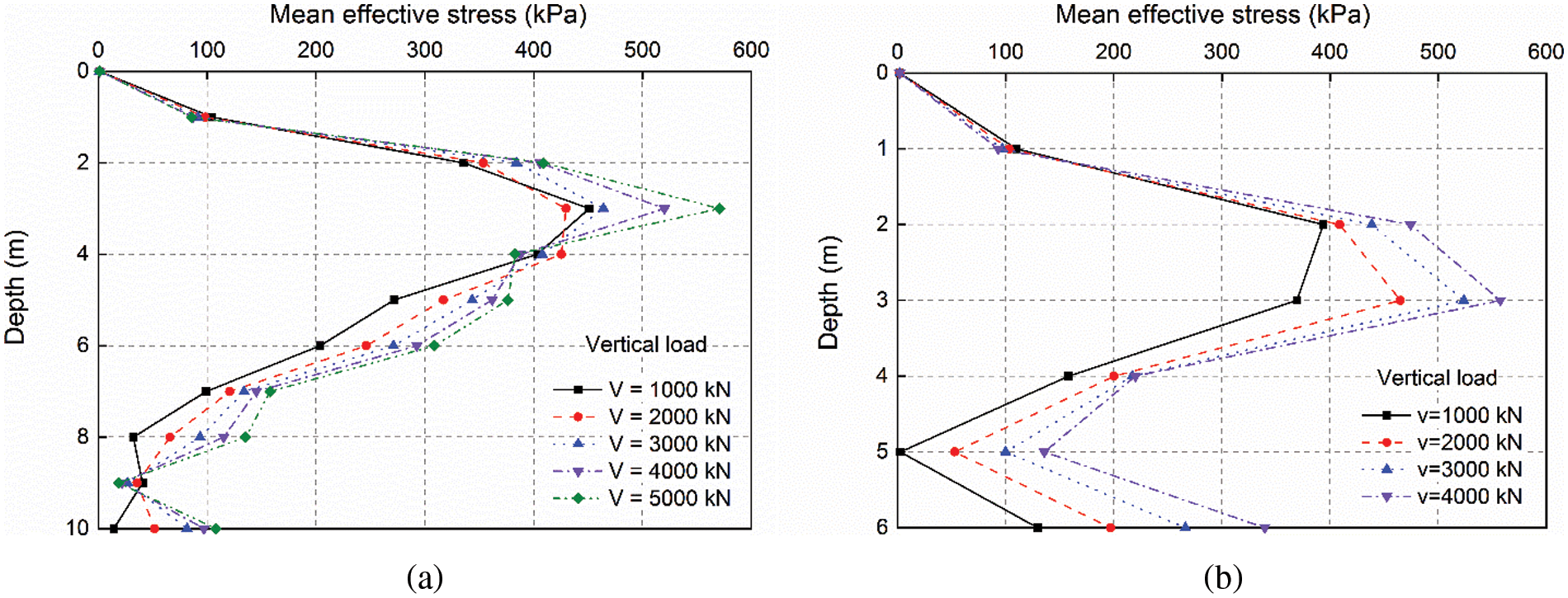Figure 7: Variation of mean effective stresses (σm) along depth of piles in sand at L = 0.1D: (a) L/D = 5 and (b) L/D = 3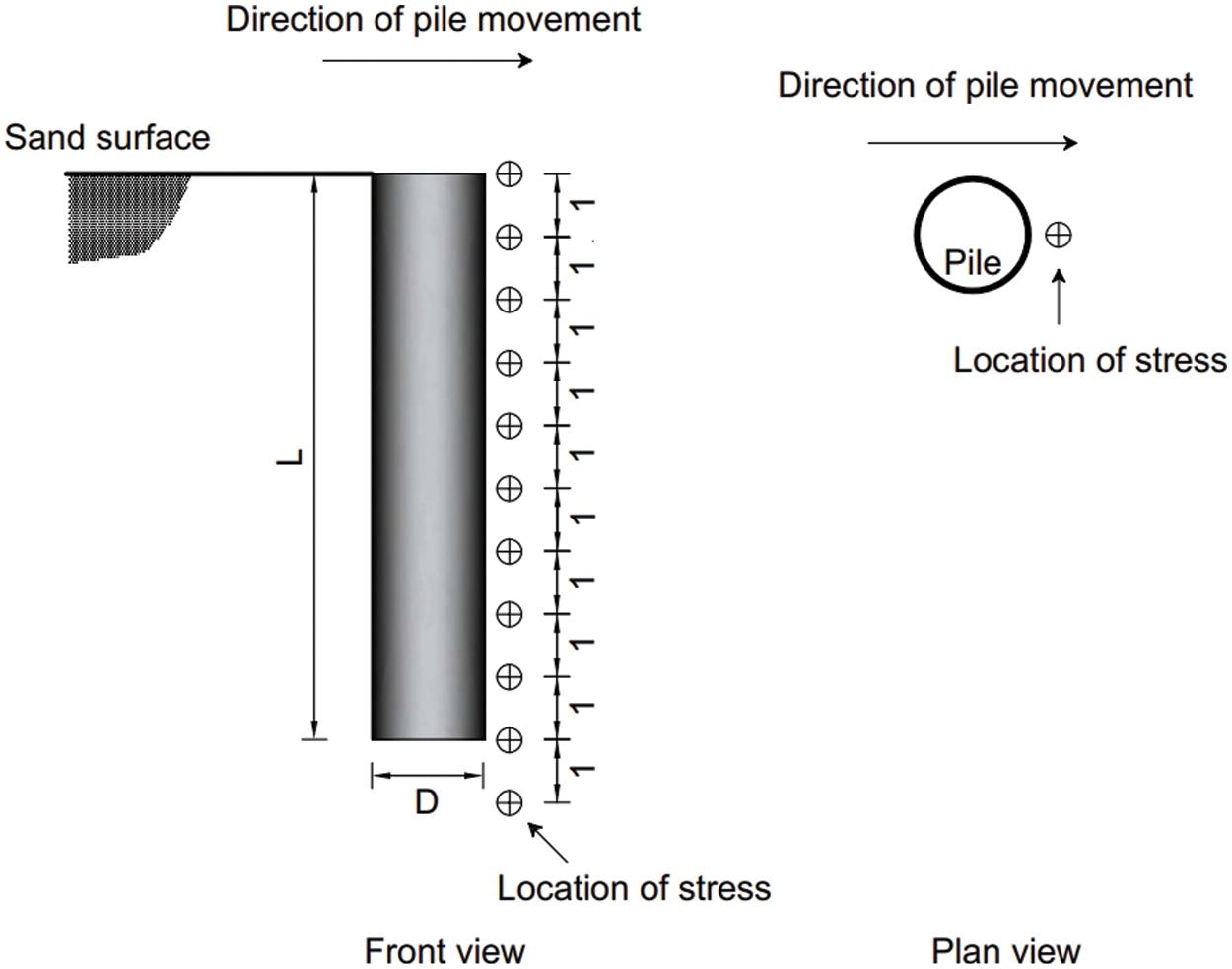Figure 8: Schematic showing stress points along the embedment depth of the pile (unit: m)

Figs. 7a and 7b present plots of the mean effective stresses (σm) in the sand in front of the pile along the pile embedment for L/D = 5 and L/D = 3, respectively, showing that mean effective stress increases with the increase of vertical load, resulting in the increase of sand stiffness.

Figs. 9a and 9b present plots of the lateral effective stresses (σxx) of the sand elements in front of the pile along the pile embedment for L/D = 5 and L/D = 3, respectively. It can be seen from the figures that the lateral effective stresses (σxx) in front of the pile were substantially higher under large vertical load, which could be be directly attributed to the increase in confining stress and sand stiffness as they could provide larger lateral stress along the frictional face. These findings are consistent with those obtained by Karthigeyan et al. .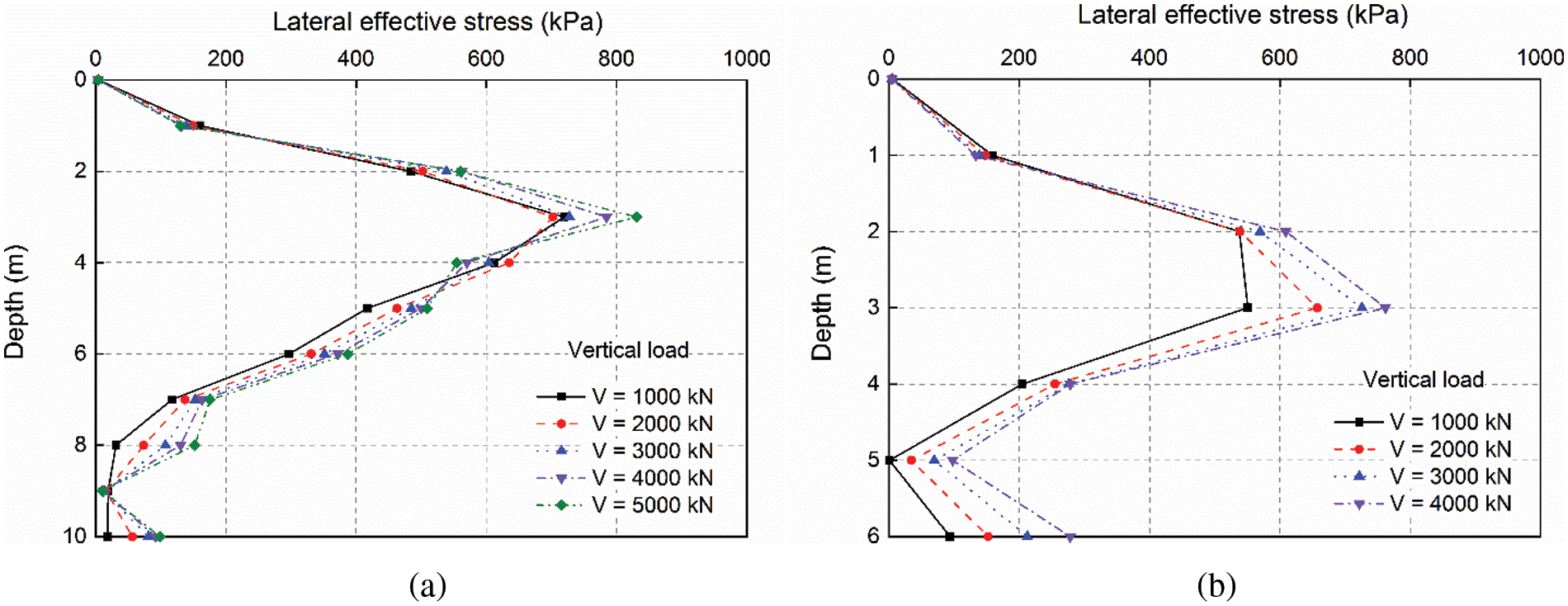Figure 9: Variation of lateral effective stresses (σxx) along depth of piles in sand at L = 0.1D: (a) L/D = 5 and (b) L/D = 3

The increase in mean effective stresses (Δσm) caused by vertical load in the shallow sand layers was further illustrated by the shadings plots on the XZ-plane, as shown in Figs. 10a and 10b for the piles with L/D ratios of 5 and 3, respectively. Herein, Δσm was calculated by using mean effective stresses under vertical load of 2 MN to subtract the mean effective stress when the pile was under the initial condition (under zero vertical load). These shadings were extracted at the Y coordinate of 0.01 m. It is clearly seen that the mean effective stress of sand around the pile was higher in the presence of vertical load than that of both the piles under zero vertical load.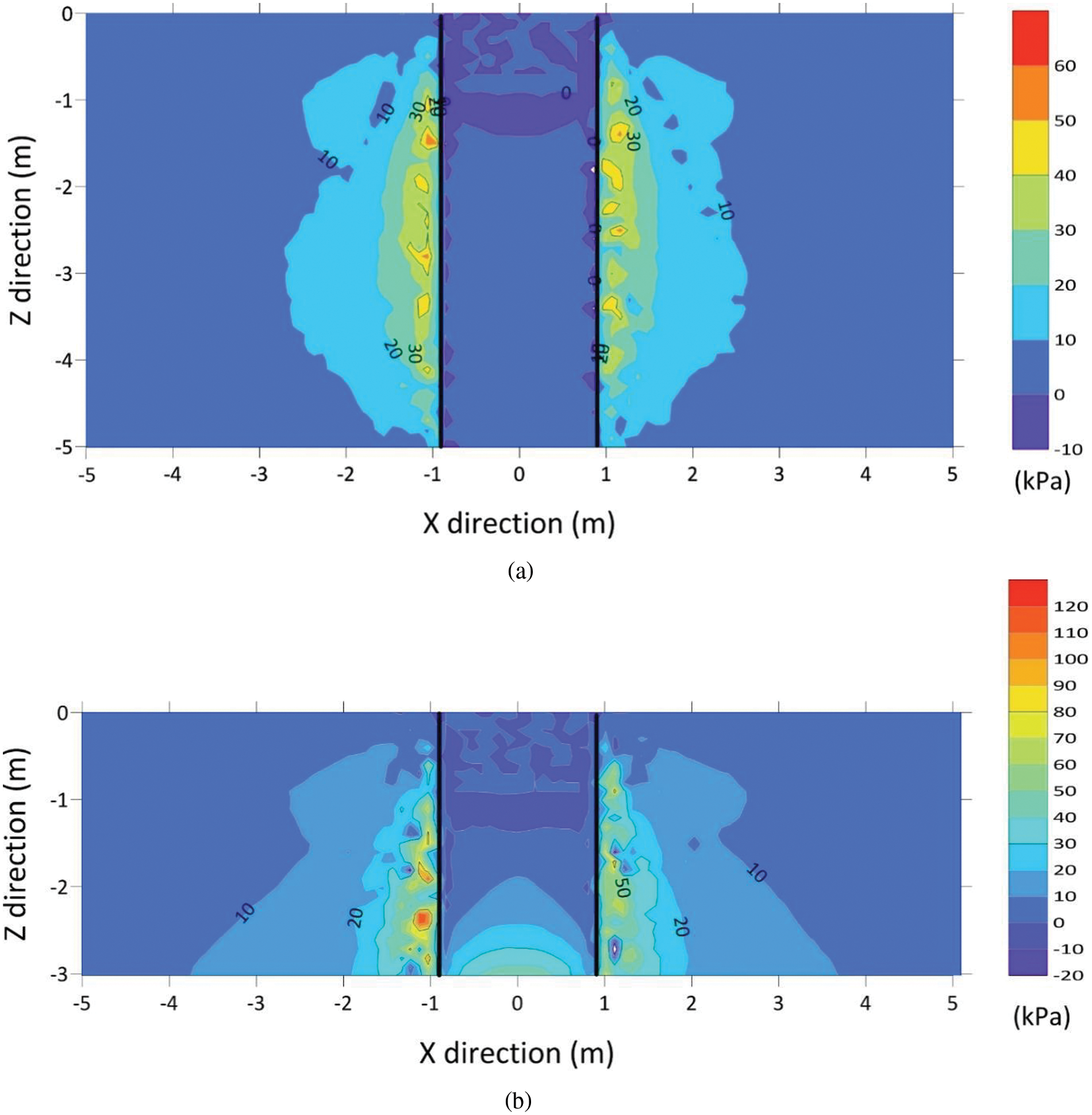Figure 10: Increase of mean effective stress on the XZ-plane by applying vertical load of 2 MN: (a) L/D = 5 and (b) L/D = 3

Fig. 11 shows the increase in mean effective stresses (Δσm) caused by vertical load on the XY-plane at the depth of 1.5D (2.7 m) for the piles with L/D ratios of 5 (Fig. 11a) and 3 (Fig. 11b), respectively. As can be clearly seen from the figures, the mean effective stress of sand around the pile was higher in the presence of vertical load. It is noticeable that under the same amount of vertical load, the increase of mean effective stresses (Δσm) on the pile with L/D ratio of 3 was about twice that for pile with L/D ratio of 5 due to the much smaller pile embedment.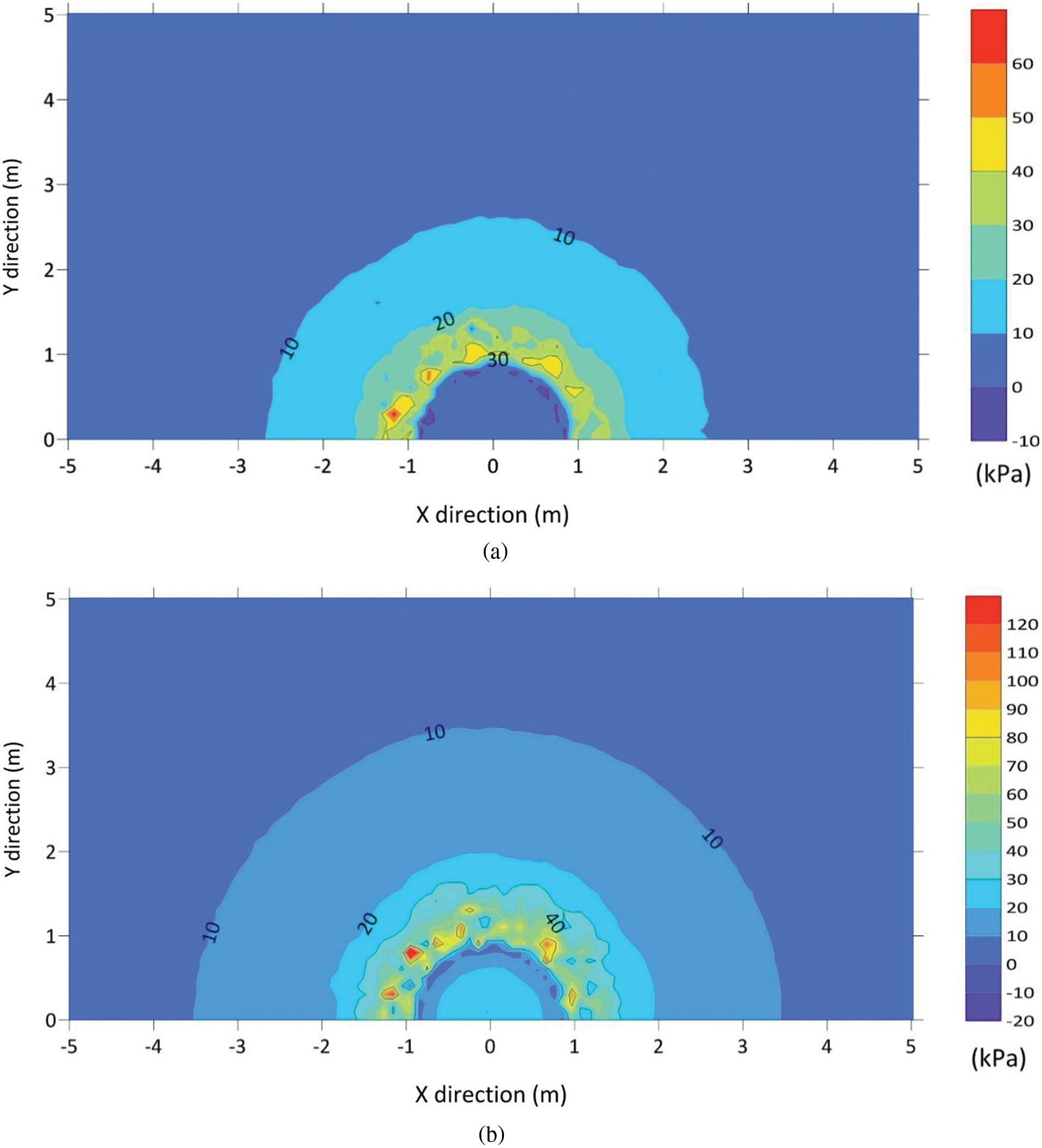Figure 11: Increase of mean effective stress on the XY-plane by applying vertical load of 2 MN: (a) L/D = 5 and (b) L/D = 3 (at depth z = 1.5D)

4  Conclusions

The effect of vertical load on the combined load response of monopiles in marine sand is an important subject for correctly evaluating the performance of the offshore foundation. Three-dimensional numerical analyses were conducted on piles under different loading sequences and with varying L/D ratios in this study. The following conclusions are drawn from the numerical results.

In the presence of vertical load, the lateral deflection of the pile in the VPL and SAVL cases decreases while it increases in the LPV case. Similar results were observed for piles with L/D ratios of 3, 5 and 8. Taking the pile with an L/D ratio of 5 for example, under increased vertical load, the lateral capacity increases considerably in the VPL case (in the order of 13% to 45%), increases marginally in the SAVL case (in the order of 12% to 30%), but decreases marginally in the LPV case (in the order of −0.4% to −10%). The reason for increased lateral capacity under the action of applied vertical load in the common practical application case, namely, the VPL case, was examined by investigating the variation law in soil stress along the depth of the pile. The confining effect by vertical load increases means effective stresses in the soil around the pile, thus increasing soil stiffness and pile capacity.

When comparing the influence of L/D ratio on the lateral load-deflection behaviour under combined load, a similar trend was found among the three L/D ratios. Piles with a smaller embedment (L/D ratio) are more sensitive to the change of vertical load amplitude in the VPL and SAVL cases. In the LPV case, the effect of the slenderness ratio is not significant. In the VPL case, piles with L/D ratios of 3, 5 and 8 experience a decline in the lateral deflection by 75%, 56% and 35% respectively under the vertical load of V = Vult compared to that without vertical load. In the SAVL case, piles with L/D ratios of 3, 5 and 8 witness a decline in the lateral deflection by 50%, 27% and 8% respectively under the applied vertical load of V = Vult compared to that without vertical load. However, in the LPV case, the lateral deflection of piles with L/D ratios of 3, 5 and 8 increases by 43%, 43% and 36% respectively under the vertical load of V = Vult compared to that without vertical load.

The results in this paper suggest that the sequence of load application governs the subsequent deformation development of monopiles. It should be noted, however, that the loading sequences applied in this paper are physically inadmissible in practice, and thus in that sense, it is not appropriate to simultaneously apply the vertical and lateral load incrementally (as in the SAVL case). The application of this approach in this work shows that not only load combination and magnitude, but loading sequence needs to be properly modelled when investigating the effect of combined load on pile. A future study is planned to experimentally validate the findings of this paper.

Funding Statement: This work is funded by the section of Geo-Engineering, Delft University of Technology.

Conflicts of Interest: The authors declare that they have no conflicts of interest to report regarding the present study.

References

1.  1.  Wu, X., Hu, Y., Li, Y., Yang, J., Duan, L. et al. (2019). Foundations of offshore wind turbines: A review. Renewable and Sustainable Energy Reviews, 104, 379–393. DOI 10.1016/j.rser.2019.01.012.
2.  2.  Choo, Y. W., Kim, D., Park, J. H., Kwak, K., Kim, J. H. et al. (2014). Lateral response of large-diameter monopiles for offshore wind turbines from centrifuge model tests. Geotechnical Testing Journal, 37(1), 107–120. DOI 10.1520/GTJ20130081.
3.  3.  Premalatha, M., Abbasi, T., Abbasi, S. (2014). Wind energy: Increasing deployment, rising environmental concerns. Renewable and Sustainable Energy Reviews, 31, 270–288. DOI 10.1016/j.rser.2013.11.019.
4.  4.  Prendergast, L. J., Gavin, K., Doherty, P. (2015). An investigation into the effect of scour on the natural frequency of an offshore wind turbine. Ocean Engineering, 101, 1–11. DOI 10.1016/j.oceaneng.2015.04.017.
5.  5.  Gavin, K., Igoe, D., Doherty, P. (2011). Piles for offshore wind turbines: A state of the art review. Proceedings of the ICE-Geotechnical Engineering, 164(4), 245–256. DOI 10.1680/geng.2011.164.4.245.
6.  6.  Europe, W. (2017). The European offshore wind industry-Key trends and statistics 2016. Brussels, Belgium: Wind Europe.
7.  7.  Li, Q., Prendergast, L. J., Askarinejad, A., Gavin, K. (2020). Influence of vertical loading on behavior of laterally-loaded foundation piles: A review. Journal of Marine Science and Engineering, 8(12), 1029. DOI 10.3390/jmse8121029.
8.  8.  Klein, G., Karavaev, V. (1979). Design of reinforced-concrete piles for vertical and horizontal loading. Soil Mechanics and Foundation Engineering, 16(6), 321– 324. DOI 10.1007/BF01710323.
9.  9.  Madhav, M., Sarma, C. (1982). Analysis of axially and laterally loaded long piles. Proceedings 2nd International Conference on Offshore Pilings, pp. 577–596. Austin, Texas.
10. 10. Trochanis, A. M., Bielak, J., Christiano, P. (1991). Three-dimensional nonlinear study of piles. Journal of Geotechnical Engineering, 117(3), 429–447. DOI 10.1061/(ASCE)0733-9410(1991)117:3(429).
11. 11. Abdel-Rahman, K., Achmus, M. (2006). Numerical modeling of the combined axial and lateral loading of vertical piles. 6th European Conference on Numerical Methods in Geotechnical Engineering, Graz, Austria.
12. 12. Karthigeyan, S., Ramakrishna, V., Rajagopal, K. (2006). Influence of vertical load on the lateral response of piles in sand. Computers and Geotechnics, 33(2), 121–131. DOI 10.1016/j.compgeo.2005.12.002.
13. 13. Karthigeyan, S., Ramakrishna, V., Rajagopal, K. (2007). Numerical investigation of the effect of vertical load on the lateral response of piles. Journal of Geotechnical and Geoenvironmental Engineering, 133(5), 512–521. DOI 10.1061/(ASCE)1090-0241(2007)133:5(512).
14. 14. Achmus, M., Thieken, K. (2010a). On the behavior of piles in non-cohesive soil under combined horizontal and vertical loading. Acta Geotechnica, 5(3), 199–210. DOI 10.1007/s11440-010-0124-1.
15. 15. Achmus, M., Thieken, K. (2010b). Behavior of piles under combined lateral and axial loading. In: Frontiers in offshore geotechnics II, pp. 465–470. Perth, Australia.
16. 16. Haiderali, A., Cilingir, U., Madabhushi, G. (2013). Lateral and axial capacity of monopiles for offshore wind turbines. Indian Geotechnical Journal, 43(3), 181–194. DOI 10.1007/s40098-013-0056-4.
17. 17. Taheri, O., Moayed, R. Z., Nozari, M. (2015). Lateral soil-pile stiffness subjected to vertical and lateral loading. Journal of Geotechnical and Transportation Engineering, 1, 30–37.
18. 18. Brinkgreve, R., Kumarswamy, S., Swolfs, W. (2015). Plaxis 3D anniversary edition manual. Plaxis bv. The Netherlands Delft. https://www.plaxis.com/support/manuals/plaxis-3d-manuals.
19. 19. Mu, L., Kang, X., Feng, K., Huang, M., Cao, J. (2018). Influence of vertical loads on lateral behaviour of monopiles in sand. European Journal of Environmental and Civil Engineering, 22(sup1), 286–301. DOI 10.1080/19648189.2017.1359112.
20. 20. Schanz, T., Vermeer, P. A., Bonnier, P. G. (2019). The hardening soil model: formulation and verification. In: Beyond 2000 in computational geotechnics, pp. 281–296. Abingdon: Routledge.
21. 21. Brinkgreve, R., Kumarswamy, S., Swolfs, W. (2018). PLAXIS material models manual 2018. https://www.plaxis.com/support/manuals/plaxis-3d-manuals.
22. 22. Brinkgreve, R., Engin, E., Engin, H. (2010). Validation of empirical formulas to derive model parameters for sands. In: Numerical methods in geotechnical engineering, 1st Edition. CRC Press.
23. 23. Lee, J. (2008). Experimental investigation of the load response of model piles in sand. West Lafayette: Purdue University.
24. 24. Arany, L., Bhattacharya, S., Macdonald, J., Hogan, S. (2017). Design of monopiles for offshore wind turbines in 10 steps. Soil Dynamics and Earthquake Engineering, 92, 126–152. DOI 10.1016/j.soildyn.2016.09.024.
25. 25. Li, Q., Gavin, K., Askarinejad, A., Prendergast, L. J. (2022). Experimental and numerical investigation of the effect of vertical loading on the lateral behaviour of monopiles in sand. Canadian Geotechnical Journal, 59(5), 652–666. DOI 10.1139/cgj-2020-0769.

Li, Q., Chen, P., Gao, L., Meng, D., Zou, J. (2023). Influence of Vertical Load on Lateral-Loaded Monopiles by Numerical Simulation. CMES-Computer Modeling in Engineering & Sciences, 135(1), 465–481.This work is licensed under a Creative Commons Attribution 4.0 International License , which permits unrestricted use, distribution, and reproduction in any medium, provided the original work is properly cited.

View

• ### 0

Like

Related articles
• Magwede K, MP Tshisikhawe, D Luseba,...
• Martínez-Corral L, E Martínez-Rubín,...
• DARÍO C. COLAUTTI, MAURICIO REMES...